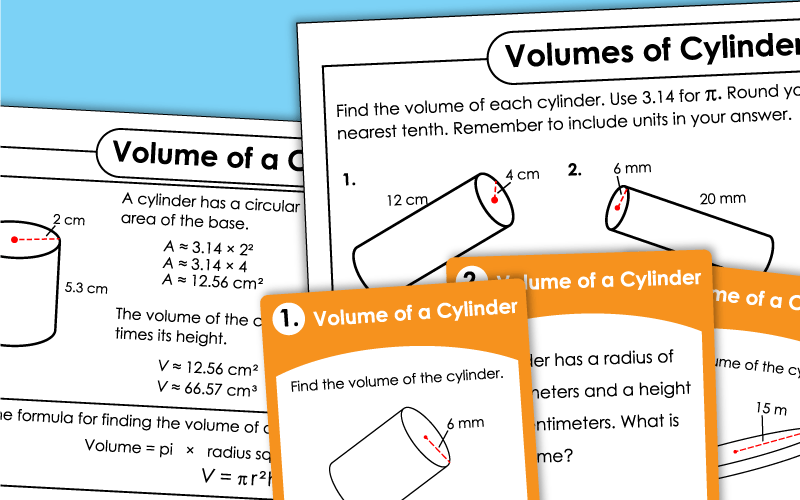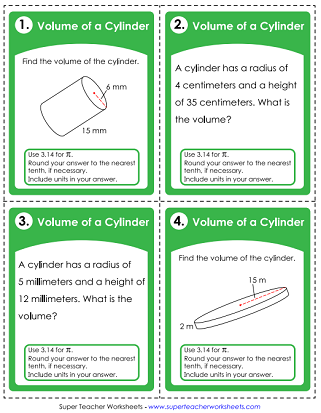# Volume of a Cylinder

The worksheets below can be used to help teach students how to calculate the volumes of cylinders. The basic level worksheets do not include decimal measurements. The advanced level includes decimals and/or fractional measurements.## Level: Basic

Calculate the volume of each cylinder. There is an example and formula at the top of the page.
This worksheet shows 9 cylinders. The height and radius of the bases are given. Students calculate the volume of each.
Compare the volumes of two cylinders. Answer 14 questions that walk you through volume calculations step-by-step.
This file has 30 task cards that you can cut apart and use for small group instruction, peer study groups, learning centers, classroom scavenger hunts, and classroom games.

The top of this page is an explanation of how to find the volume of a cylinder. Beneath that are six problems for students to solve.
Calculate the volume of 9 different cylinders. In this set, one or more of the measurements given is a decimal.
Solve each word problem by finding volumes of cylinders. Problems include decimals up to hundredths.
Volumes of Rectangular Prisms

## Sample Worksheet ImagesMy Account
Site Information